## Complete the square

### Quick description

When faced with a expression involving both purely quadratic terms (e.g.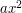) and linear terms (e.g.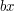) in one or more variables, translate the variablein order to absorb the linear terms into the quadratic ones. This can create additional constant terms, but all other things being equal, constant terms are preferable to deal with than linear terms.

### Prerequisites

High school algebra

### Example 1: The quadratic formula

This is the classic example of the completing the square trick. Suppose one wants to find all solutions to the quadratic equation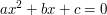where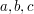are parameters. The linear term prevents one from solving this equation directly; however, observe that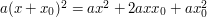for any shift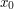. Thus, (assuming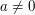) if one picks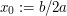, one can absorb the linear factor into the quadratic one, obtaining an equationwhich only involves quadratic and constant terms. Now, the rules of high school algebra can solve this equation. Dividing byand rearranging, one gets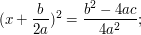taking square roots, we arrive at the famous formula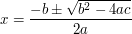for the two solutions to the quadratic equation, bearing in mind that the square root may well be imaginary if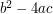is negative. (For the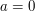, the above formula does not make sense, but in that case the equation degenerates to the linear equation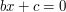, which has one solution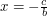, unlessmanaged to degenerate to zero also, in which case there are no solutions when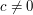and an infinite number of solutions when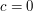.)

See "how to solve quadratic equations" for more discussion.

### Example 2

A quadratic form is an expression like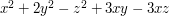. That particular example is a quadratic form on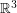, and in general a quadratic form over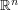is a functionobtained by taking a symmetric bilinear form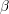onand defining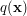to be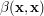. In the example above, the bilinear form is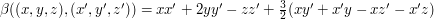.

It is often convenient to diagonalize a quadratic form, which means writing it as a linear combination of squares of linearly independent linear forms. Let us do this for the example above, by completing the square.

Our aim will be to achieve this by removing fromthe square of a linear form in such a way thatis no longer involved. To do this, we first pick out the terms that involve, which are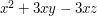. We then try to find a linear form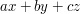such that when you square it the terms involvingare precisely these ones. With a bit of experience in completing the square, we know that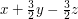will do the job. Indeed,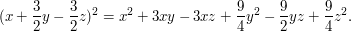Therefore,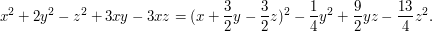We would now like to finish this process by diagonalizing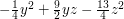, which we can make look slightly nicer by writing it as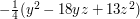. Completing the square again, we find that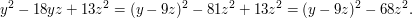Plugging this in, we deduce that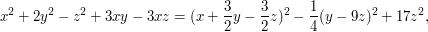and the quadratic form has been diagonalized.

### Example 3

Completing the square can be used to compute the Fourier transform of gaussians. In one dimension, this was done in "Use rescaling or translation to normalize parameters"; we do the multi-dimensional case here. Specifically, let us compute the Fourier transform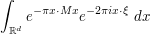(1)

of the Gaussian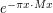, where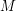is a positive definite real symmetric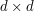matrix, and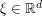. Observe that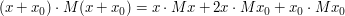for all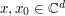. Thus, if we pick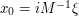, we can complete the square and rewrite (1) as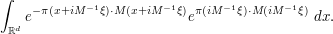The constant term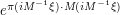, being independent of, can be pulled out of the integral and simplified, leaving us with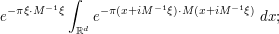contour shifting in each of the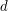variables of integration separately then allows us to rewrite this as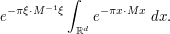Making the change of variables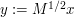to normalize the integrand, we can simplify this as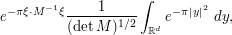which by factoring the integral and using the standard integral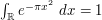(proven at square and rearrange) simplifies to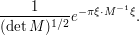Remark Of course, one could also go about this computation by diagonalizingfirst to place it in a normal form; it is instructive to see how both computations end up at the same answer at the end of the day.

### Example 4

The standard proof of the Cauchy-Schwarz inequality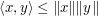in a Hilbert space (which we take here to be real, for simplicity) can be viewed as a variant of the completing-the-square trick, but now one converts linear term into quadratic and constant terms rather than vice versa. Indeed, since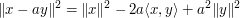for any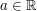, we can write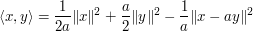for any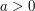; in particular,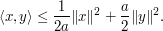If we then optimize in, we obtain the Cauchy-Schwarz inequality.

### Example 5

Suggestions welcome!

### General discussion

Completing the square can also be done in several variables, whenever one is adding a quadratic form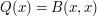to a linear form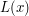plus some constant terms, provided that the quadratic form is non-degenerate (this is analogous to thecondition in the quadratic formula).

The method also works to some extent for higher degree polynomials, but is significantly weaker. For instance, with cubic equations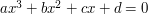, one can complete the cube to eliminate the quadratic factor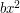(at the expense of modifying the lower order terms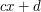), but the linear term remains, and further tricks are needed to solve this equation.

### Possible further examples

I have a couple of suggestions. One is to do a Gaussian computation in this article. A natural example is to take a bivariate normal random variable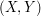and create out of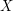and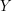two linear combinations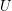and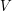that are linearly independent. That should perhaps come after my second suggestion, which is simply to explain how to diagonalize a quadratic form (by giving a few examples). If I have a spare moment, I may add these examples.

The second one is one I care about because a lot of people I teach seem to have the impression that the only way of diagonalizing a quadratic form is to diagonalize the associated symmetric matrix.

Incidentally, I'm all in favour of using the same example in more than one article, so there might be a case for having the Fourier transform of a Gaussian done here as well.

### completing the square to prove inequalities

I've seen this used multiple times, in a context similar to the usual proof of Cauchy-Schwarz inequality, where completing the square of a real-valued expression shows that some grouping of terms is non-negative.

### Thanks for the suggestions!

I put up some quick examples to reflect them. Feel free to edit, of course.

The following comments were made inline in the article. You can click on 'view commented text' to see precisely where they were made.

### Contour shifting

I've started an article about making complex substitutions in integrals. My guess is that this is what you mean by contour shifting, but I have not put this link in yet because I am not quite sure. If it is, and if this is the standard name for the technique, then I'll add a remark to that effect to the article.

### Contour shifting

Hmm, I thought this was a common term, but I just googled for it and the first mathematical occurrence of it was... one of my own web pages. But "shifting the contour" is a reasonably common term. It's not _quite_ the same as complex substitution (it's based on Cauchy's theorem for contour integrals, rather than the change of variables formula), but it of course combines very well with such substitutions. But it also can be used independently of complex substitution, e.g. to evaluate integrals such as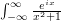by shifting the contour to a big semicircle etc.

I link to this nonexistent article in a couple other places, so I think I'll start a stub on it, and perhaps return to it later.

How can it be possible to transform the method of completing the square into such a "charabia".

By the way, a(x+x_0)^2 = ax^2 + 2ax x_0 + x_0^2 is false.

### I don't find the article

I don't find the article unintelligible at all; perhaps you could clarify what you find problematic.

Also, editing a page to correct typos (like the missing coefficient you spotted) is very simple: just click on edit at the top of the article. I've corrected it here.

## Post new comment

(Note: commenting is not possible on this snapshot.)

Before posting from this form, please consider whether it would be more appropriate to make an inline comment using the Turn commenting on link near the bottom of the window. (Simply click the link, move the cursor over the article, and click on the piece of text on which you want to comment.)